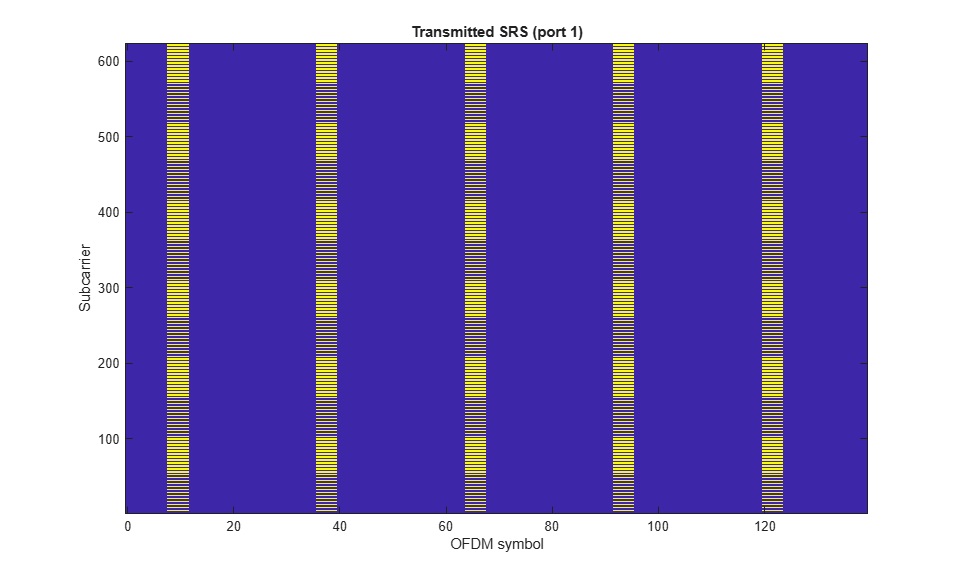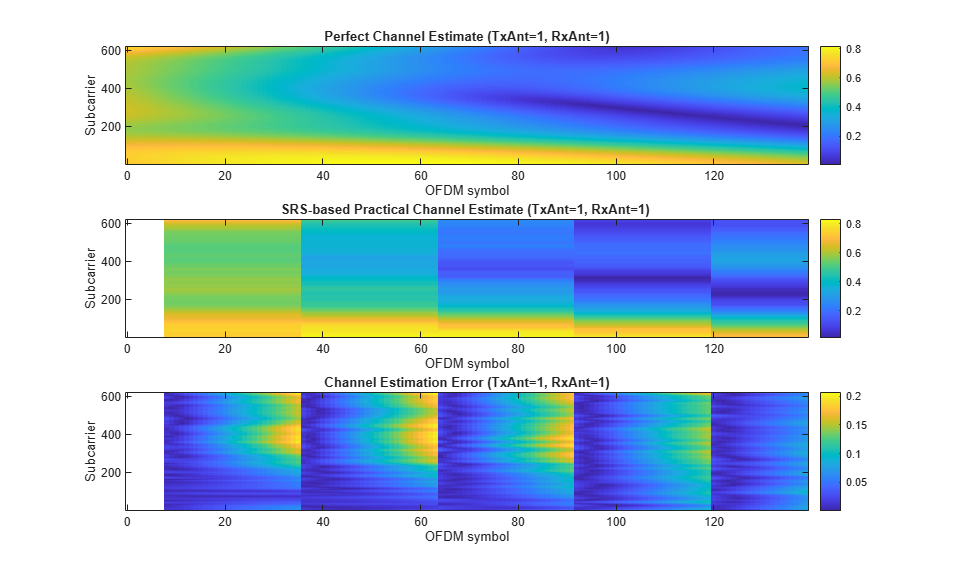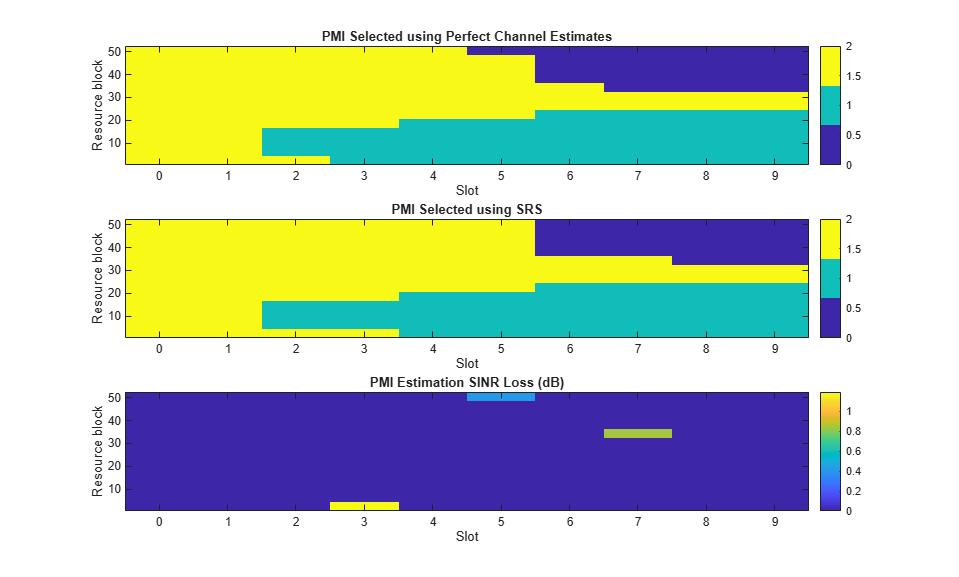# NR Uplink Channel State Information Estimation Using SRS

This example shows how to use sounding reference signals (SRS) for synchronization, channel estimation, and uplink channel state information (CSI) estimation.

### Introduction

Sounding reference signals are uplink physical signals used by user equipment (UE) for uplink channel sounding, including synchronization and CSI estimation. CSI comprises channel quality indicator (CQI), rank indicator (RI), and precoder matrix indicator (PMI). This example demonstrates how to use SRS to select the appropriate PMI under frequency-selective time-varying noisy channels. Uplink codebook-based transmissions use PMIs, as defined in TS 38.211 Section 6.3.1.5 [ 1 ].

This example performs a simulation including:

• SRS configuration and transmission

• Perfect and practical synchronization and channel estimation

• Signal-to-noise ratio (SNR) estimation

• PMI selection

• PMI selection performance assessment

### Simulation Length and SNR

Set the length of the simulation in terms of the number of 10 ms frames. Set the SNR points to simulate. The SNR is defined per RE and applies to each receive antenna.

```numFrames = 1; % 10 ms frames snr = 20; % SNR in dB ```

### UE and SRS Configuration

Set the key parameters of the simulation. These include:

• The bandwidth in resource blocks (12 subcarriers per resource block)

• Subcarrier spacing: 15, 30, 60, 120, 240 (kHz)

• Cyclic prefix length: normal or extended

• Number of transmit and receive antennas: 1, 2 or 4.

• Number of layers. It must be lower than or equal to the number of transmit and receive antennas.

The SRS parameters specified include:

• Number of SRS antenna ports: 1,2,4

• Number of OFDM symbols allocated for SRS per slot: 1,2,4

• Starting OFDM symbol of the SRS transmission within a slot. It must be (8...13) for normal CP and (6...11) for extended CP.

• Starting position of the SRS in frequency specified in RBs

• Bandwidth and frequency hopping configuration `CSRS`, `BSRS`, and `BHop`. Set `BHop >= BSRS` to disable frequency hopping.

• Transmission comb to specify the SRS frequency density in subcarriers: 2,4

• Number of repeated SRS symbols within a slot. It disables frequency hopping in blocks of `Repetition` symbols. Set `Repetition = 1` for no repetition.

• Periodicity and offset of the SRS in slots.

• Resource type can be 'periodic', 'semi-persistent', and 'aperiodic'. The frequency hopping pattern is reset for aperiodic SRS resource types in every slot.

```% Create UE/carrier configuration ue = nrCarrierConfig; ue.NSizeGrid = 52; % Bandwidth in number of resource blocks (52RBs at 15kHz SCS for 10MHz BW) ue.SubcarrierSpacing = 15; % 15, 30, 60, 120, 240 (kHz) ue.CyclicPrefix = 'Normal'; % 'Normal' or 'Extended' nTxAnts = 2; % Number of transmit antennas (1,2,4) nRxAnts = 2; % Number of receive antennas nLayers = min(nTxAnts,nRxAnts); % Configure a periodic multi-port SRS and enable frequency hopping srs = nrSRSConfig; srs.NumSRSSymbols = 4; % Number of OFDM symbols allocated per slot (1,2,4) srs.SymbolStart = 8; % Starting OFDM symbol within a slot srs.NumSRSPorts = nTxAnts; % Number of SRS antenna ports (1,2,4). srs.FrequencyStart = 0; % Frequency position of the SRS in BWP in RBs srs.NRRC = 0; % Additional offset from FreqStart specified in blocks of 4 PRBs (0...67) srs.CSRS = 14; % Bandwidth configuration C_SRS (0...63). It controls the allocated bandwidth to the SRS srs.BSRS = 0; % Bandwidth configuration B_SRS (0...3). It controls the allocated bandwidth to the SRS srs.BHop = 0; % Frequency hopping configuration (0...3). Set BHop < BSRS to enable frequency hopping srs.KTC = 2; % Comb number (2,4). Frequency density in subcarriers srs.Repetition = 2; % Repetition (1,2,4). It disables frequency hopping in blocks of |Repetition| symbols srs.SRSPeriod = [2 0]; % Periodicity and offset in slots. SRSPeriod(2) must be < SRSPeriod(1) srs.ResourceType = 'periodic'; % Resource type ('periodic', 'semi-persistent','aperiodic'). Use 'aperiodic' to disable inter-slot frequency hopping ```

### Synchronization, Channel Estimation and CSI Measurement Configuration

This example performs synchronization and channel estimation in SRS candidate slots. Timing and channel estimates are updated only in slots containing SRS transmissions. In frequency-hopping SRS setups, channel estimates are only updated in those resource blocks containing SRS symbols. When there is no SRS transmission, timing and channel estimates from previous slots are held and used for CSI acquisition. Similarly, noise power estimates are updated only in SRS candidate slots.

The logical variable `practicalSynchronization` controls channel synchronization behavior. When set to `true`, the example performs practical synchronization based on the values of the received SRS. When set to `false`, the example performs perfect synchronization. Synchronization is performed only in slots where the SRS is transmitted to keep perfect and practical channel estimates synchronized.

```practicalSynchronization = true; ```

This example estimates CSI by splitting the carrier bandwidth into a number of subbands. Specify the size of the frequency subbands in RB

```csiSubbandSize = 4; % Number of RBs per subband ```

### Propagation Channel Model Configuration

Create a TDL channel model object and specify its propagation characteristics. Select the channel delay spread and maximum Doppler shift to create a time-varying and frequency-selective channel within the simulation duration and carrier bandwidth.

```channel = nrTDLChannel; channel.DelayProfile = 'TDL-C'; channel.DelaySpread = 40e-9; channel.MaximumDopplerShift = 30; channel.NumTransmitAntennas = nTxAnts; channel.NumReceiveAntennas = nRxAnts; channel.Seed = 5; % Set channel sample rate ofdmInfo = nrOFDMInfo(ue); channel.SampleRate = ofdmInfo.SampleRate; % Get the maximum delay of the channel chInfo = info(channel); maxChDelay = chInfo.MaximumChannelDelay; % Reset random generator for reproducibility rng('default'); ```

### Processing Loop

Measure the CSI per slot. The CSI is obtained using the following steps:

• Generate resource grid. Use SRS symbols and indices to create a resource element (RE) grid.

• Generate waveform. The generated grid is then OFDM modulated.

• Model noisy channel. The waveform is passed through a TDL fading channel. AWGN is added. The SNR for each layer is defined per RE and per receive antenna.

• Perform synchronization and OFDM demodulation. For perfect synchronization, the channel impulse response is reconstructed and used to synchronize the received waveform. For practical synchronization, the received waveform is correlated with the SRS. The synchronized signal is then OFDM demodulated.

• Perform channel estimation. For perfect channel estimation, the channel impulse response is reconstructed and OFDM demodulated to provide a channel estimate. For practical channel estimation, the transmitted SRS is used.

• PMI selection. The SRS-based channel estimate is used to select the best PMI in each CSI estimation subband. The PMI selection criterion maximizes the average signal-to-interference-plus-noise-ratio (SINR) after precoding.

• PMI selection SINR loss. The SINR loss is calculated by comparing the SINR after precoding with the estimated and ideal PMIs. Ideal PMIs are selected using perfect channel estimates.

```% Number of slots to simulate numSlots = numFrames*ue.SlotsPerFrame; % Total number of subcarriers and symbols per slot K = ue.NSizeGrid * 12; L = ue.SymbolsPerSlot; % Initialize arrays storing channel estimates allTxGrid = zeros([K L*numSlots nTxAnts]); slotGridSize = [K L nRxAnts nTxAnts]; hEst = zeros(slotGridSize); hestInterp = zeros(slotGridSize); hEstUpdate = zeros(slotGridSize); totalGridSize = [K L*numSlots nRxAnts nTxAnts]; allHest = zeros(totalGridSize); allHestPerfect = zeros(totalGridSize); allHestInterp = zeros(totalGridSize); % Initialize noise power estimate nvar = 0; % Calculate the number of CSI subbands for the carrier numCSISubbands = ceil(ue.NSizeGrid/csiSubbandSize); % Initialize SINR per subband, slot, and PMI maxPMI = hMaxPUSCHPrecodingMatrixIndicator(nLayers,nTxAnts); sinrSubband = zeros([numCSISubbands numSlots maxPMI+1]); % Initialize PMI matrix and SINR loss pmi = NaN(numCSISubbands,numSlots); pmiPerfect = pmi; loss = zeros(size(pmi)); % Initialize timing estimation offset offset = chInfo.ChannelFilterDelay; % Calculate SRS CDM lengths cdmLengths = hSRSCDMLengths(srs); % OFDM symbols used for CSI acquisition csiSelectSymbols = srs.SymbolStart + (1:srs.NumSRSSymbols); for nSlot = 0:numSlots-1 % Update slot counter ue.NSlot = nSlot; % Generate SRS and map to slot grid [srsIndices,srsIndInfo] = nrSRSIndices(ue,srs); srsSymbols = nrSRS(ue,srs); % Create a slot-wise resource grid empty grid and map SRS symbols txGrid = nrResourceGrid(ue,nTxAnts); txGrid(srsIndices) = srsSymbols; % Determine if the slot contains SRS isSRSSlot= ~isempty(srsSymbols); % OFDM Modulation [txWaveform,waveformInfo] = nrOFDMModulate(ue,txGrid); txWaveform = [txWaveform; zeros(maxChDelay, size(txWaveform,2))]; %#ok<AGROW> % required later to flush the channel filter to obtain the received signal % Transmission through channel [rxWaveform,pathGains] = channel(txWaveform); % Add AWGN to the received time domain waveform % Normalize noise power to take account of sampling rate, which is % a function of the IFFT size used in OFDM modulation. The SNR % is defined per RE for each receive antenna (TS 38.101-4). SNR = 10^(snr/10); N0 = 1/sqrt(2.0*nRxAnts*double(waveformInfo.Nfft)*SNR); noise = N0*complex(randn(size(rxWaveform)),randn(size(rxWaveform))); rxWaveform = rxWaveform + noise; % Perform timing offset estimation pathFilters = getPathFilters(channel); % Timing estimation is only performed in the slots where the SRS is % transmitted to keep the perfect and practical channel estimation % synchronized. if isSRSSlot if practicalSynchronization % Practical synchronization. Correlate the received waveform % with the SRS to give timing offset estimate offset = nrTimingEstimate(ue,rxWaveform,srsIndices,srsSymbols); else offset = nrPerfectTimingEstimate(pathGains,pathFilters); %#ok<UNRCH> end end % Perform OFDM demodulation rxGrid = nrOFDMDemodulate(ue,rxWaveform(1+offset:end,:)); % Perform practical channel estimation % Update channel estimates only in the symbols and RBs containing SRS % in this slot and hold the estimates from the previous slot in other % locations. nvar is not updated when there is no SRS transmission. Use % a time-averaging window that covers all the SRS symbols transmitted. if isSRSSlot % this slot contains an SRS transmission [hEst,nvar] = nrChannelEstimate(ue,rxGrid,srsIndices,srsSymbols,'AveragingWindow',[0 7],'CDMLengths',cdmLengths); % Use channel estimate from previous slot for OFDM symbols before the first SRS symbol hestInterp = repmat(hestInterp(:,end,:,:),1,ue.SymbolsPerSlot); % Update channel estimate in OFDM symbols and RB where the SRS is % present and hold all channel estimates until the end of the slot firstSymbol = srs.SymbolStart+1; lastSymbol = srs.SymbolStart + srs.NumSRSSymbols; hEstUpdate(:,firstSymbol:lastSymbol,:,:) = hEst(:,firstSymbol:lastSymbol,:,:); hEstUpdate(:,lastSymbol:L,:,:) = repmat(hEst(:,lastSymbol,:,:),1,ue.SymbolsPerSlot-lastSymbol+1); idxHEstUpdate = hEstUpdate ~= 0; % Indices of updated channel estimates hestInterp(idxHEstUpdate) = hEstUpdate(idxHEstUpdate); else % Hold previous channel estimates if this slot does not contain SRS hestInterp = repmat(hestInterp(:,end,:,:),1,ue.SymbolsPerSlot); end % PMI Selection % Select the precoder matrix indicators for a number of layers using % the interpolated channel estimates in the OFDM symbols specified by % csiSelectSymbols. The PMIs are estimated per CSI subband [pmi(:,nSlot+1),sinrSubband(:,nSlot+1,:),subbandIndices] = hPMISelect(nLayers, hestInterp(:,csiSelectSymbols,:,:), nvar, csiSubbandSize); % PMI selection SINR loss % Calculate the performance loss as a ratio of the SINR after precoding % with PMIs selected using a practical channel estimate and the SINR % after precoding with PMIs selected using a perfect channel estimate. % Calculate perfect channel estimate for perfect PMI selection hEstPerfect = nrPerfectChannelEstimate(ue,pathGains,pathFilters,offset); % Perfect noise estimate from noise realization noiseGrid = nrOFDMDemodulate(ue,noise(1+offset:end,:)); nvarPerfect = var(noiseGrid(:)); [loss(:,nSlot+1),pmiPerfect(:,nSlot+1)] = hPMISelectionSINRLoss(pmi(:,nSlot+1), nLayers, hEstPerfect(:,csiSelectSymbols,:,:), nvarPerfect); % Save a copy of all transmitted OFDM grids and channel estimates for % display purposes thisSlot = nSlot*L + (1:L); % Symbols of the current slot allTxGrid(:,thisSlot,:) = txGrid; allHest(:,thisSlot,:,:) = hEst; allHestInterp(:,thisSlot,:,:) = hestInterp; allHestPerfect(:,thisSlot,:,:) = hEstPerfect; end ```

### Results

This section displays these results for all configured frames:

• Transmitted OFDM grid containing SRS

• Perfect and practical channel estimates, and channel estimation error. The error is calculated as the absolute value of the difference between the perfect and practical channel estimates.

• Selected PMI using perfect and practical channel estimates, and the PMI absolute error.

• Average SINR per subband after precoding with best estimated PMI

• SINR performance loss

```% Create x-axis and y-axis vectors symbols = 0:(ue.NSlot+1)*ue.SymbolsPerSlot-1; slots = 0:ue.NSlot; subcarriers = 1:ue.NSizeGrid*12; resourceBlocks = 1:ue.NSizeGrid; ```

Display the transmitted OFDM grid containing SRS

```figure imagesc(symbols,subcarriers,abs(allTxGrid(:,:,1,1))); xlabel('OFDM symbol'); ylabel('Subcarrier'); axis xy; title('Transmitted SRS (port 1)'); ```Display perfect and practical channel estimates, and channel estimation error per OFDM symbol and subcarrier. The channel estimation error is defined as the absolute value of the difference between the perfect and practical channel estimates.

```% Remove first OFDM symbols not containing SRS to improve visualization of % channel estimation error hEstInterp = allHestInterp; idx = 1:(srs.SRSPeriod(2)*ue.SymbolsPerSlot + srs.SymbolStart); hEstInterp(:,idx,:,:) = NaN; hEstInterp((srs.NRB*12+1):end,:,:,:) = NaN; hChannelEstimationPlot(symbols,subcarriers,allHestPerfect,hEstInterp); ```Display the selected PMI using perfect and practical channel estimates, and the PMI selection SINR loss per slot and RB. The SINR loss is defined as a ratio of the SINR after precoding with estimated and perfect PMIs. Estimated PMIs are obtained using a practical channel estimate and perfect PMIs are selected using a perfect channel estimate.

```% First expand loss from subbands into RBs for display purposes pmiRB = hExpandSubbandToRB(pmi, csiSubbandSize, ue.NSizeGrid); pmiPerfectRB = hExpandSubbandToRB(pmiPerfect, csiSubbandSize, ue.NSizeGrid); lossRB = hExpandSubbandToRB(loss, csiSubbandSize, ue.NSizeGrid); hPMIPlot(slots,resourceBlocks,pmiRB,pmiPerfectRB,lossRB); ```Next, compare the PMIs obtained using both perfect and practical channel estimates. This indicates the ratio of correct to total PMIs and the location in the resource grid where errors have occurred.

```numLayers = min(size(allHestInterp,[3 4])); if numLayers ~= 1 pmiErr = sum( abs(pmi - pmiPerfect) > 0, [1 2])./ sum( ~isnan(pmi), [1 2]); TotPMIEst = sum(~isnan(pmi),[1 2]); fprintf('Number of estimated PMI: %d \n', TotPMIEst); fprintf(' Number of wrong PMI: %d \n', ceil(pmiErr*TotPMIEst)); fprintf(' Relative error: %.1f (%%) \n', pmiErr*100); else fprintf('For a single layer, PMI is always 0.\n'); end ```
```Number of estimated PMI: 130 Number of wrong PMI: 3 Relative error: 2.3 (%) ```

Display the SINR per slot and RB obtained after precoding with the PMI that maximizes the SINR per subband.

```hBestSINRPlot(slots,resourceBlocks,sinrSubband,pmi,csiSubbandSize); ```### Summary and Further Exploration

This example shows how to use SRS for synchronization, channel estimation and PMI selection commonly employed in codebook-based uplink transmission modes. The example also evaluates the channel estimation and PMI selection performance loss using the SINR after precoding.

You can investigate the performance of the channel estimation and PMI selection in more complex settings. Increase the SRS periodicity and observe how the channel estimates aging introduces a delay and worsens the SINR after precoding. In addition, you can investigate the performance under frequency hopping conditions by setting the SRS parameters BHop < BSRS. In this setup, channel estimates aging is not uniform in frequency.

### Appendix

This example uses the following helper functions:

### References

1. 3GPP TS 38.211. "NR; Physical channels and modulation" 3rd Generation Partnership Project; Technical Specification Group Radio Access Network.

2. 3GPP TS 38.101-4. "NR; User Equipment (UE) radio transmission and reception. Part 4: Performance requirements" 3rd Generation Partnership Project; Technical Specification Group Radio Access Network.

### Local functions

```% Displays perfect and practical channel estimates and the channel % estimation error for the first transmit and receive ports. The channel % estimation error is defined as the absolute value of the difference % between the perfect and practical channel estimates. function hChannelEstimationPlot(symbols,subcarriers,allHestPerfect,allHestInterp) figure subplot(311) imagesc(symbols, subcarriers, abs(allHestPerfect(:,:,1,1))); axis xy; xlabel('OFDM symbol'); ylabel('Subcarrier'); colorbar; title('Perfect Channel Estimate (TxAnt=1, RxAnt=1)'); subplot(312) imagesc(symbols, subcarriers, abs(allHestInterp(:,:,1,1)), ... 'AlphaData',~isnan(allHestInterp(:,:,1,1))) axis xy; xlabel('OFDM symbol'); ylabel('Subcarrier'); colorbar; title('SRS-based Practical Channel Estimate (TxAnt=1, RxAnt=1) '); % Display channel estimation error, defined as the difference between the % SRS-based and perfect channel estimates subplot(313) hestErr = abs(allHestInterp - allHestPerfect); imagesc(symbols, subcarriers, hestErr(:,:,1,1),... 'AlphaData',~isnan(hestErr(:,:,1,1))); axis xy; xlabel('OFDM symbol'); ylabel('Subcarrier'); colorbar; title('Channel Estimation Error (TxAnt=1, RxAnt=1)'); end % Displays the PMI evolution and PMI estimation SINR loss over time and % frequency. The SINR loss is defined as a ratio of the SINR after % precoding with estimated and perfect PMIs. Estimated PMIs are % obtained using a practical channel estimate and perfect PMIs are % selected using a perfect channel estimate. function hPMIPlot(slots,resourceBlocks,pmiRB,pmiPerfectRB,lossRB) figure subplot(311) imagesc(slots,resourceBlocks,pmiPerfectRB,'AlphaData',~isnan(pmiPerfectRB)); axis xy; c = clim; cm = colormap; colormap( cm(1:floor(size(cm,1)/(c(2)-c(1)) -1):end,:) ); % Adjust colormap to PMI discrete values colorbar xlabel('Slot'); ylabel('Resource block'), title('PMI Selected using Perfect Channel Estimates') subplot(312) imagesc(slots,resourceBlocks,pmiRB,'AlphaData',~isnan(pmiRB)); axis xy; colorbar, xlabel('Slot'); ylabel('Resource block'), title('PMI Selected using SRS') subplot(313) imagesc(slots,resourceBlocks,lossRB,'AlphaData',~isnan(lossRB)); colormap(gca,cm) xlabel('Slot'); ylabel('Resource block'); axis xy; colorbar; title('PMI Estimation SINR Loss (dB)') end % Displays the SINR per resource block obtained after precoding with the % PMI that maximizes the SINR per subband. function hBestSINRPlot(slots,resourceBlocks,sinrSubband,pmi,csiBandSize) % Display SINR after precoding with best PMI bestSINRPerSubband = nan(size(sinrSubband,[1 2])); % Get SINR per subband and slot using best PMI [sb,nslot] = find(~isnan(pmi)); for i = 1:length(sb) bestSINRPerSubband(sb(i),nslot(i)) = sinrSubband(sb(i),nslot(i),pmi(sb(i),nslot(i))+1); end % First expand SINR from subbands into RBs for display purposes bestSINRPerRB = hExpandSubbandToRB(bestSINRPerSubband, csiBandSize, length(resourceBlocks)); figure sinrdb = 10*log10(abs(bestSINRPerRB)); imagesc(slots,resourceBlocks,sinrdb,'AlphaData',~isnan(sinrdb)); axis xy; colorbar; xlabel('Slot'); ylabel('Resource block') title('Average SINR Per Subband and Slot After Precoding with Best PMI (dB)') end % Expands a 2D matrix of values per subband in the first dimension into a % matrix of values per resource block. function rbValues = hExpandSubbandToRB(subbandValues, bandSize, NRB) lastBandSize = mod(NRB,bandSize); lastBandSize = lastBandSize + bandSize*(lastBandSize==0); rbValues = [kron(subbandValues(1:end-1,:),ones(bandSize,1));... subbandValues(end,:).*ones(lastBandSize,1)]; end ```